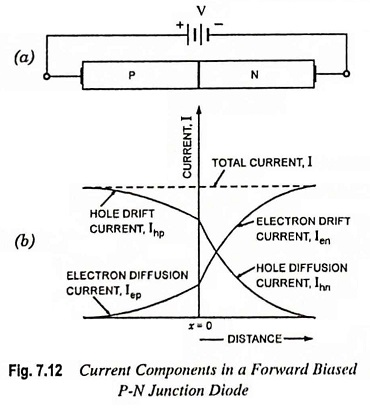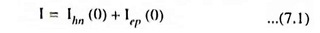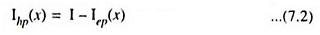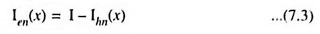## Current Components in PN Junction Diode:

A Current Components in PN Junction Diode with a forward bias with an external voltage V is shown in Fig. 7.12 (a). Due to forward bias, there exists a potential gradient in P and N materials. Now the holes from P-region and electrons from N-region are injected into the N-region and P-region respectively. The holes injected into the N-region and electrons injected into the P-region are the minority carriers. The minority carriers diffuse away from the junction exponentially with the distance, as illustrated in Fig. 7.12 (b). Their concentration falls steadily due to recombination with electrons and holes respectively. Since the diffusion current due to minority carriers is proportional to the concentration gradient, this current must also vary exponentially with distance. There are two minority charge carrier (diffusion) currents, Iep and Ihn as shown in Fig. 7.12 (b). Let the symbols Iep (x) and Ihn (x) represent the electron current in P-region and hole current in N-region respectively.Electrons crossing the junction at x = 0 from right to left constitute a current in the same direction as the holes crossing the junction from left to right. Thus the total current I at junction (i.e., x = 0) is given asSince the current is the same throughout a series circuit, total current I is independent of x, and is shown as a horizontal line in Fig. 7.12 (b). So, in the P-region there must be a second component of current Ihp which combining with Iep gives the total current I. So the majority carrier (or hole) current, Ihp in the P-region is given asSimilarly the majority (or electron) current, Ien in the N-region is given asThe currents Ihp and Ien are plotted as a function of distance in Fig. 7.12 (b). The Fig. 7.12 (b) is drawn for unsymmetrically doped diode, so that Iep ≠ Ihn.

It may be noted that far away from the junction in P-region the current is drift current Ihp of holes sustained by small electric field in the semiconductor. As the holes approach the junction, some of them recombine with the electrons, which have been injected into the P-region from the N-region. Thus part of the current Ihp becomes a negative current just equal in magnitude to the diffusion current Iep. The drift current Ihp, therefore, decreases toward the junction. The rate of decrease is such that the total current remains constant, independent of distance. The reduced hole current at the junction now enters the N-region and becomes hole diffusion current Ihn. Similar remarks can be made with respect to electron drift current in N-region, Ien. Thus in a forward-biased P-N diode, the current enters the P-region as a hole current and leaves the N-region as an electron current of the same magnitude.

From the above discussion it is concluded that the Current Components in PN Junction Diode is bipolar in character (because it is made up of both positive and negative charge carriers) and the total current is constant throughout the device, but the proportion of current due to holes and that due to electrons varies with the distance, as illustrated in Fig. 7.12 (b).

Scroll to Top